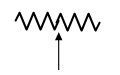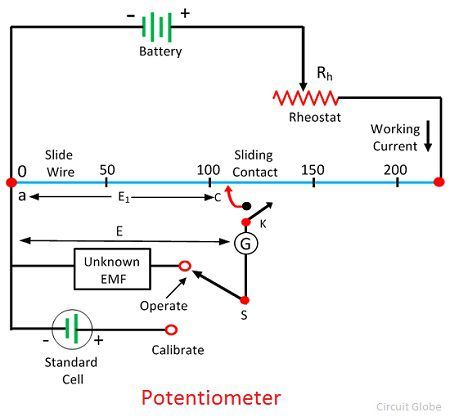# Difference Between Potentiometer & Voltmeter

The potentiometer and the voltmeter both are the voltage measuring device. The significant difference between the two is that the potentiometer measures the emf of the circuit whereas voltmeter measures the end terminal voltage of the circuit. The other differences between the potentiometer and the voltmeter are explained below in the comparison chart.

The potentiometer works on the principle of the comparison method. It compares the unknown voltage sources by the standard voltage source. When their voltages are equal, the galvanometer shows the null deflection. The voltmeter is connected in parallel with the circuit whose voltage is to be measured.

Content: Potentiometer Vs Voltmeter

## Comparison Chart

Basis For Comparison Potentiometer Voltmeter
Definition It is an instrument used for measuring the unknown voltage.Type of meter designs for measuring the voltage.
SymbolMeasure Value Accurately Approximately
Sensitivity High Low
Instrument Type Null Deflection TypeDeflection Type
ResistanceInfinite Very high but measurable

### Definition of Potentiometer

The potentiometer is the three terminal device used for measuring the unknown voltage source by comparing it with the known voltage. The standard source supplies the known voltage.

The potentiometer has seven thin wires place parallels to each other. The one end of the wire is attached to each other with the help of the copper strips. The copper strips convert the seven parallel wires into one. The battery and the variable resistor is attached in parallel with the emf source.The one end of the unknown and known source is connected in parallel with each other. The other end of the sources is connected to the two way switch. The galvanometer is connected to the other end of the switch. The jockey which is connected to the galvanometer is moved on the wire until the galvanometer shows the null deflection.

### Definition of Voltmeter

The voltmeter measures the voltages between any two points of an electrical circuit. The voltmeter has high internal resistance because of which the meter draws small current.

The working of the voltmeter depends on the high internal resistance. The voltmeter is of two types, i.e., the analogue or digital voltmeter. It is always connected in parallel with the circuit.The current through the measurand source passes through the high resistance circuit of the meter. In analogue voltmeter, the value of the unknown voltage is known by the product of the resistance, and the current flows through the circuit.

## Key Differences Between Potentiometer and Voltmeter

The following are the key differences between potentiometer and voltmeter.

1. The potentiometer is an instrument used for measuring the emf, whereas the voltmeter is a type of meter which measures the terminal voltage of the circuit.
2. The potentiometer accurately measures the potential difference because of zero internal resistance. Whereas, the voltmeter has a high internal resistance which causes the error in measurement. Thus the voltmeter approximately measures the voltage.
3. The sensitivity of the potentiometer is very high, i.e. it can measure small potential differences between the two points. The voltmeter has low sensitivity.
4. The potentiometer uses the null deflection type instrument whereas the voltmeter uses the deflection type instrument.
5. The potentiometer has infinite internal resistance, whereas the potentiometer has high measurable resistance.

### Conclusion

The potentiometer and voltmeter both measures the emf in volts. The potentiometer is used in a circuit where the accurate value of voltage is required. For approximate calculation, the voltmeter is used.